Function Repository Resource:

# CesaroEquation

Compute the Cesàro equation for a planar curve

Contributed by: Wolfram Staff (original content by Alfred Gray)
 ResourceFunction["CesaroEquation"][c,t,{κ,s}] computes the Cesàro equation for curvature κ and arc length s of a planar curve c parametrized by t.

## Examples

### Basic Examples (3)

Cesàro equation for the circle:

 In:=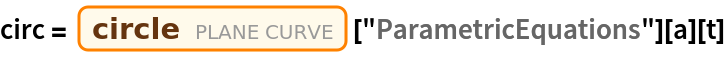Out=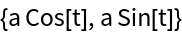In:=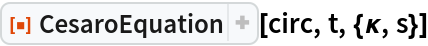Out=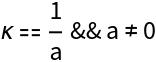Cesàro equation for the logarithmic spiral:

 In:=Out=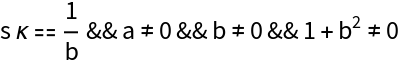Equations can be simplified using assumptions:

 In:=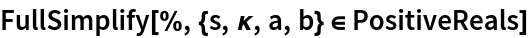Out=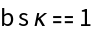Cesàro equations for several curves:

 In:=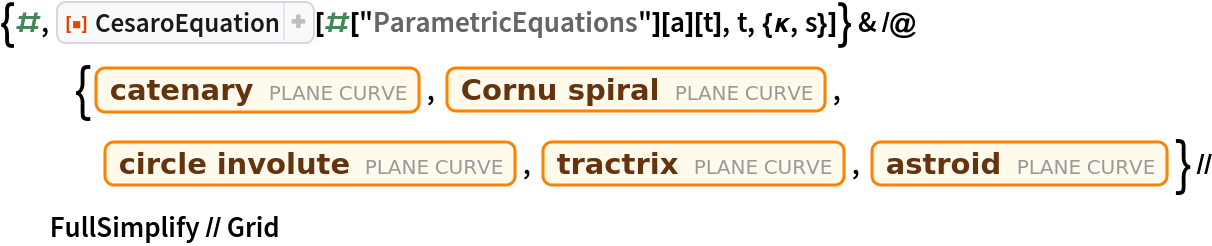Out=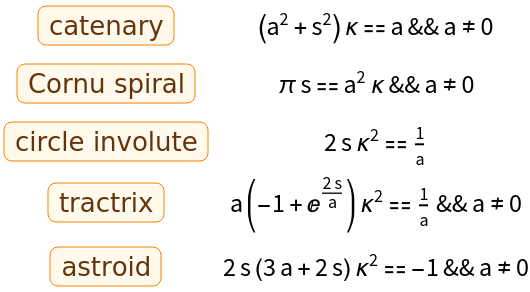### Properties and Relations (2)

For some curves, entities can be used to get the Cesàro equation:

 In:=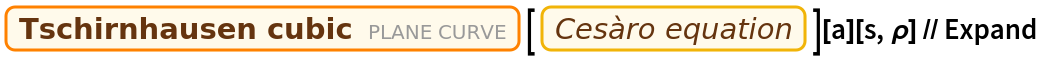Out=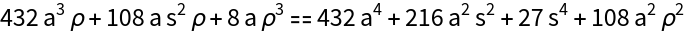This can instead be computed by CesaroEquation with κ=1/ρ:

 In:=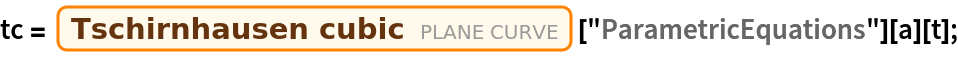In:=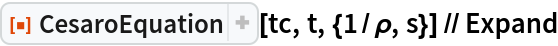Out=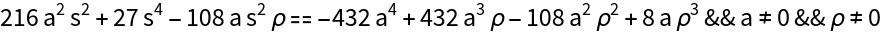Cesàro equation for the Cornu spiral:

 In:=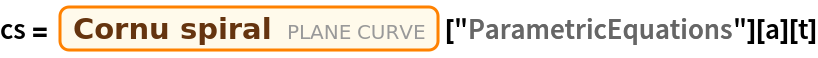Out=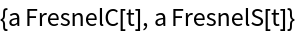In:=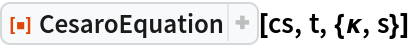Out=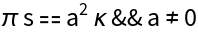Curvature is linear:

 In:=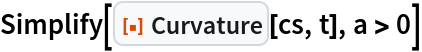Out=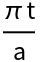Alfred Gray’s generalization of the Cornu spiral:

 In:=The clothoid for n, a both set to unity gives the Cornu spiral:

 In:=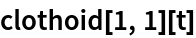Out=Plot the clothoid:

 In:=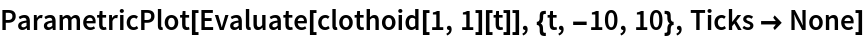Out=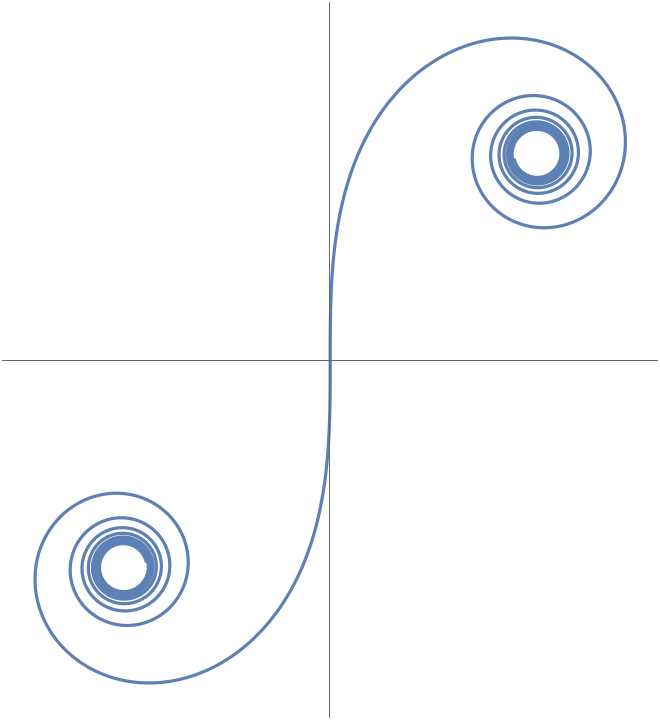Different values give other types of special functions:

 In:=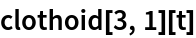Out=The clothoid for general values n, a:

 In:=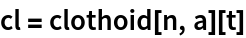Out=Find the Cesàro equation for the clothoid:

 In:=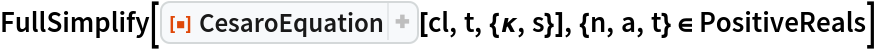Out=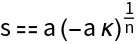The curvature takes the simpler form:

 In:=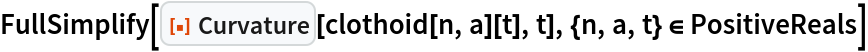Out=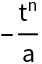A plot of quadratic intrinsic curvature:

 In:=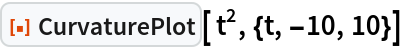Out=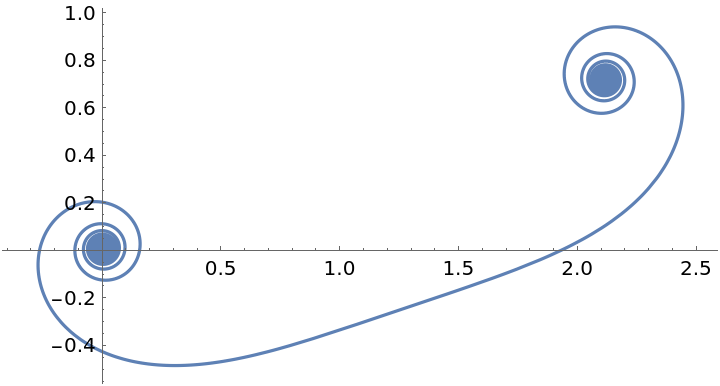Enrique Zeleny

## Version History

• 1.0.0 – 01 June 2020